## Notation

### Symbols

 Symbol Meaning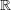set of real numbers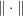norm of the argument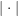modulus of the argument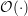Landau symbol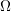unit spherecolatitude (polar angle)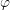longitude (azimuth)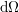unit sphere surface element,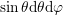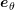,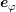unit vectors w.r.t.and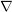Nabla operator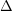Laplace operator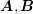matrices (capital letters, bold)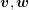vectors (lowercase, bold)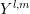real valued spherical harmonic of major indexand minor index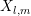spherical harmonics expansion coefficient to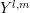of the quantity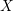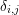Kronecker delta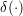delta distribution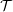triangulation of the simulation domain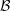Voronoi box grid of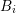a box from the adjoint box grid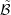dual box grid of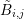a box from the box gridoverlappingand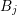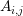interface area between boxes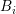and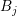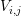volume fraction of the boxesandassociated with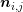surface unit normal vector of boxpointing into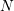number of degrees of freedom in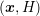-space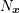number of degrees of freedom in-space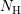number of discrete total energies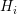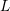spherical harmonics expansion order

 Symbol Meaning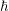Planck constant divided by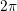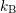Boltzmann constantpermittivity (not to be confused with kinetic energy)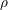space charge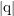elementary charge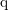charge of a carrier (negative for electrons, positive for holes)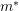effective masskinetic energy (not to be confused with permittivity)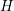total energytimespatial coordinate within the device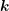wave vectormomentum vector(group) velocity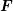force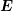electric field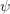quasi-electrostatic potential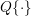scattering operator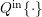in-scattering operator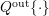out-scattering operator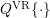velocity-randomizing scattering operatorindex of the scattering process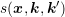scattering coefficient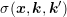symmetric scattering coefficient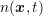particle density (electron density)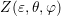generalized density of states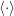moment of the BTE with respect to the argument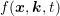distribution function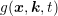generalized energy distribution functiongeneralized current density term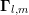spherical harmonics coupling term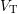thermal voltage

### Abbreviations

 BTE Boltzmann transport equation CPU central processing unit GPU graphics processing unit FET field effect transistor MEDS maximum entropy dissipation scheme MOSFET metal-oxide-semiconductor field effect transistor SHE spherical harmonics expansion TCAD technology computer aided design